Difference Between Array and Pointer

The main difference between array and pointer is that an array is a data structure that stores a collection of elements of the same data type while a pointer is a variable that holds the address of another variable in the computer memory.

Array and pointer are two concepts used in programming languages such as C and C++. An array is a collection of data that holds a fixed number of values of the same data type.  For example, to store the marks of 10 students, the programmer can create an array that can store 10 integers. On the other hand, a pointer is a variable that holds the address of another variable.  Pointers help to access memory and to manipulate memory addresses.

Key Areas Covered

1. What is an Array
– Definition, Functionality
2. What is a Pointer
– Definition, Functionality
3. Relationship Between Array and Pointer
– Outline of Association
3. Difference Between Array and Pointer
– Comparison of Key Differences

Key Terms

Array, Pointer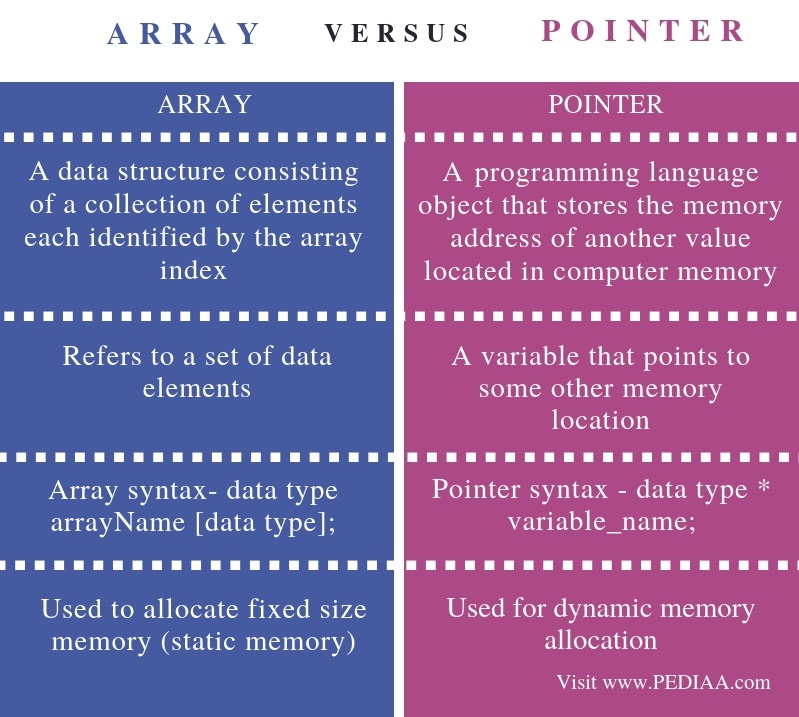What is an Array

An array is a data structure that stores a fixed number of elements of the same type. All the elements of the array are in contiguous memory locations.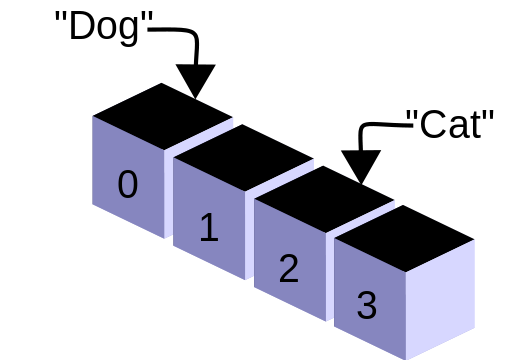Figure 1: Array

Assume that you need to store values of 50 integers. It is possible to create variables for each integer but it is difficult and more time-consuming. An array can be used to overcome this issue.

int numbers;

The above statement will allocate an array called numbers, which can store fifty integers. A specific element of the array can be assessed using the index. The starting index is 0. As there are 50 elements in the array, the final element has the index 49.

numbers = 30;

The above statement will assign value 30 to the 1st index of the numbers array.

Moreover, it is possible to create arrays of other data types.

For example, the double average; will create an array called average. It can store 10 double values.

An array is easier to create and easier to access elements. On the other hand, an array has a fixed size. If the array size is 10, it cannot be used to store 20 elements. In other words, the memory allocation for an array is static.

What is a Pointer

A variable is a memory location to store a value. Every memory location has an address to identify it.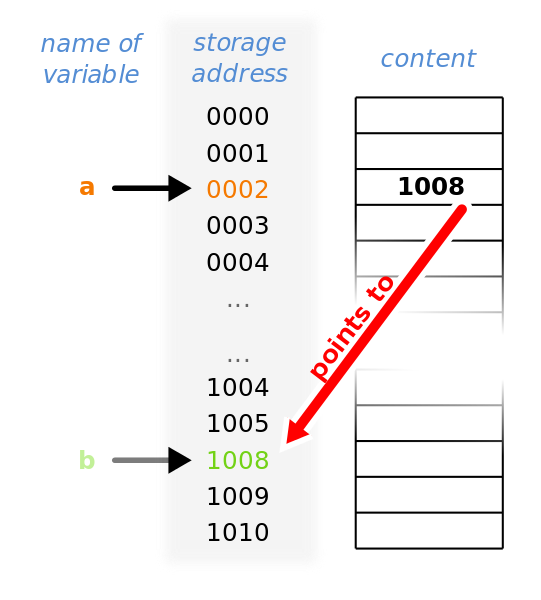Figure 2: Pointer

A pointer is a variable that stores the address of another variable. The pointer is declared before storing a variable address. The asterisk * is used to declare a pointer.

int *ptr;

The ptr is a pointer to an integer variable.

double *ptr1;

The ptr1 is a pointer to a double variable. Refer the following code.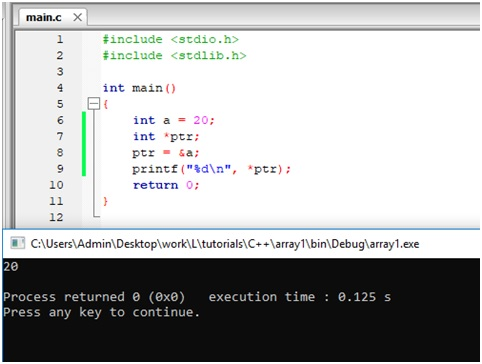Figure 3: Program with Pointers

The variable ‘a’ contains the value 20. The ptr is a pointer to an integer. In line 8, ptr is assigned with the address of variable a. Now ptr is pointing to a. Therefore, printing the value pointed by ptr will give the value of a.

If there is no address to be assigned, the pointer can be assigned with a NULL value. This type of pointer is called the null pointer.

int *ptr = NULL;

Relationship Between Array and Pointer

Refer the below program.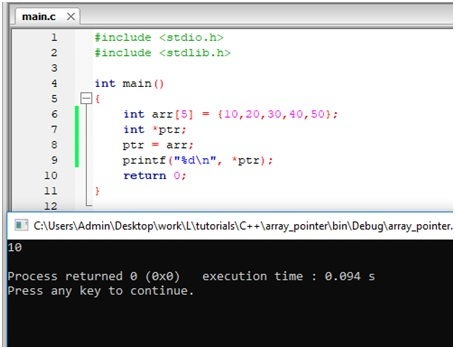Figure 4: Arrays with Pointers

There is an array called arr. It contains 5 integers. The ptr is a pointer to an integer. In line 8, arr is assigned to ptr. Therefore, the ptr is pointing to the 0th index element of the array. Printing the value of ptr will display the value of 0th index element. It is 10.

Difference Between Array and Pointer

Definition

Array is a data structure consisting of a collection of elements each identified by the array index. A pointer is a programming language object that stores the memory address of another value located in computer memory.

Basis

While an array refers to a set of data elements, a pointer is a variable that points to some other memory location.

Syntax

Array syntax – data type arrayName [data type];

Pointer syntax – data type * variable_name;

Usage

Although arrays can be used to allocate fixed size memory (static memory), pointers can be used for dynamic memory allocation.

Conclusion

The two terms array and pointer are two programming concepts. The difference between array and pointer is that an array is a data structure that stores a collection of elements of same data type while a pointer is a variable that holds the address of another variable in the computer memory.

Reference:

1. “Arrays in C.” Www.tutorialspoint.com, Tutorials Point, Available here.
2. “Pointers in C.” Www.tutorialspoint.com, Tutorials Point, Available here.

Image Courtesy:

1. “CPT-programming-array” By Pluke – Own work (CC0) via Commons Wikimedia
2. “Pointers” By This file was made by User: SvenTranslation Own work (CC BY-SA 3.0) via Commons Wikimedia